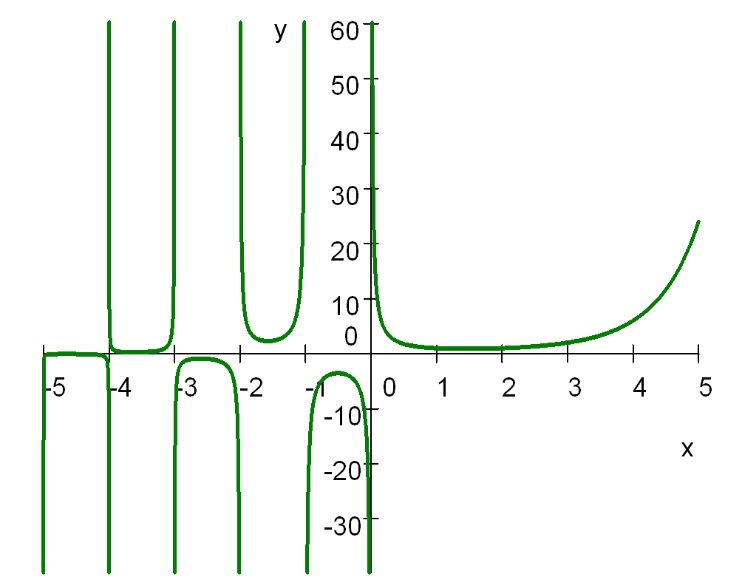## Two Mathematics Stack Exchange Questions and Answers on the Gamma Function

I post here together two answers of mine on MSE both on the gamma function.

Question by pomme. Definition of the gamma function

“I know that the Gamma function with argument$(-\dfrac{1}{ 2})$ — in other words$\Gamma(-\dfrac{1}{2})$ is equal to$-2\pi^{1/2}$. However, the definition of$\Gamma(k)=\displaystyle\int_0^\infty t^{k-1}e^{-t}dt$

but how can$\Gamma(-\frac{1}{2})$ be obtained from the definition? WA says it does not converge…”

Your doubt makes sense if for$k=-1/2$ you try using the definition of the gamma function by the integral you have written, because it diverges at$k=-1/2$ as you stated. I will try to clarify if as follows. This integral representation of the gamma function (I use$x$ instead of$k$)$\Gamma (x)=\displaystyle\int_{0}^{\infty }t^{x-1}e^{-t}dt\qquad(0)$

holds in the reals if and only if$x>0$. Using integration by parts we can show that$\Gamma (x+1)=x\Gamma (x).\qquad(1)$

For$x<0$ we can define$\Gamma (x)$ for all negative values of$x<0$ except$-1,-2,-3,\cdots$ not by the integral$(0)$rather by means of the functional equation$(1)$ in the form$\Gamma (x)=\dfrac{\Gamma(x+1)}{x}.\qquad(2)$

Then$x+1>0$ [and]$\Gamma (x+1)$ is convergent. In your example$x=-1/2$, so$x+1=1/2$ and$\Gamma (1+x)=\Gamma (1/2)$. Hence we obtain$\Gamma (-1/2)=\dfrac{\Gamma(1/2)}{-1/2}=-2\Gamma (1/2)=-2\sqrt{\pi },\qquad(3)$

where we use the known value of the integral$\Gamma(1/2)=\sqrt{\pi }$, which can be evaluated, e.g. from the equality$\displaystyle\int_{0}^{\infty }\displaystyle\int_{0}^{\infty }e^{-x^{2}-y^{2}}dxdy=\dfrac{\pi }{4}=\left( \displaystyle\int_{0}^{\infty }e^{-x^{2}}dx\right) ^{2}.\qquad(4)$

Similarly, for$x=-3/2$ by$(2)$ we find$\Gamma (-3/2)=-\frac{2}{3}\Gamma (-1/2)$, using$(3)$ twice. This process is called analytic continuation, but its true understanding requires knowledge of complex analysis.$\text{Plot of }y=\Gamma(x)\quad -5

Question by Amitabh Udayiman. Convergence of this integral

“My statistics text book prescribed by my school states that the integral$\Gamma(n)=\displaystyle\int_{0}^{\infty}e^{-x}x^{n-1}dx$

is convergent for$n>0$.It does not prove the statement. So can anyone please help me prove it? Thanks again!”

My answer. I assume that$n$ is a real number. Split the gamma improper integral$\Gamma(n)=\displaystyle\int_{0}^{\infty}e^{-x}x^{n-1}dx\qquad(0)$

into$I_1+I_2$, where$I_1=\displaystyle\int_{0}^{1}e^{-x}x^{n-1}dx\qquad(1)$

and$I_2=\displaystyle\int_{1}^{\infty}e^{-x}x^{n-1}dx\qquad(2)$

1. To prove that the integral$I_2$ is always convergent use the fact that for any real number$\alpha$ the integral$\int_{1}^{\infty }e^{-x}x^{\alpha }dx\qquad(3)$

is convergent, by the limit comparison test$\displaystyle\lim_{x\rightarrow \infty }\dfrac{e^{-x}x^{\alpha }}{x^{-2}}=0\qquad(4)$

with the convergent integral$\displaystyle\int_{1}^{\infty }\dfrac{dx}{x^{2}}.\qquad(5)$

2. As for$I_1$ consider two cases. (a) If$n\geq 1$ observe that$\lim_{x\rightarrow 0}e^{-x}x^{n-1}=0$, so$I_1$ is a proper integral. (b) If$0, the integrand$e^{-x}x^{n-1}$ behaves like$x^{n-1}$ near$n=0$, because$e^{-x}\rightarrow 1$ as$x\rightarrow 0$. Since$\displaystyle\int_{0}^{1}\dfrac{dx}{x^{1-n}}\qquad(6)$

is convergent if and only if$1-n<1$, i.e.$n>0$, so is$I_1$. It follows that$\Gamma(n)=I_1+I_2$ is convergent for$n>0.$## Sobre Américo Tavares

eng. electrotécnico reformado / retired electrical engineer
Esta entrada foi publicada em Cálculo, Função Gama, Funções Especiais, Integrais, Integrais impróprios, Matemática, Math, Mathematics Stack Exchange com as etiquetas , , . ligação permanente.

This site uses Akismet to reduce spam. Learn how your comment data is processed.